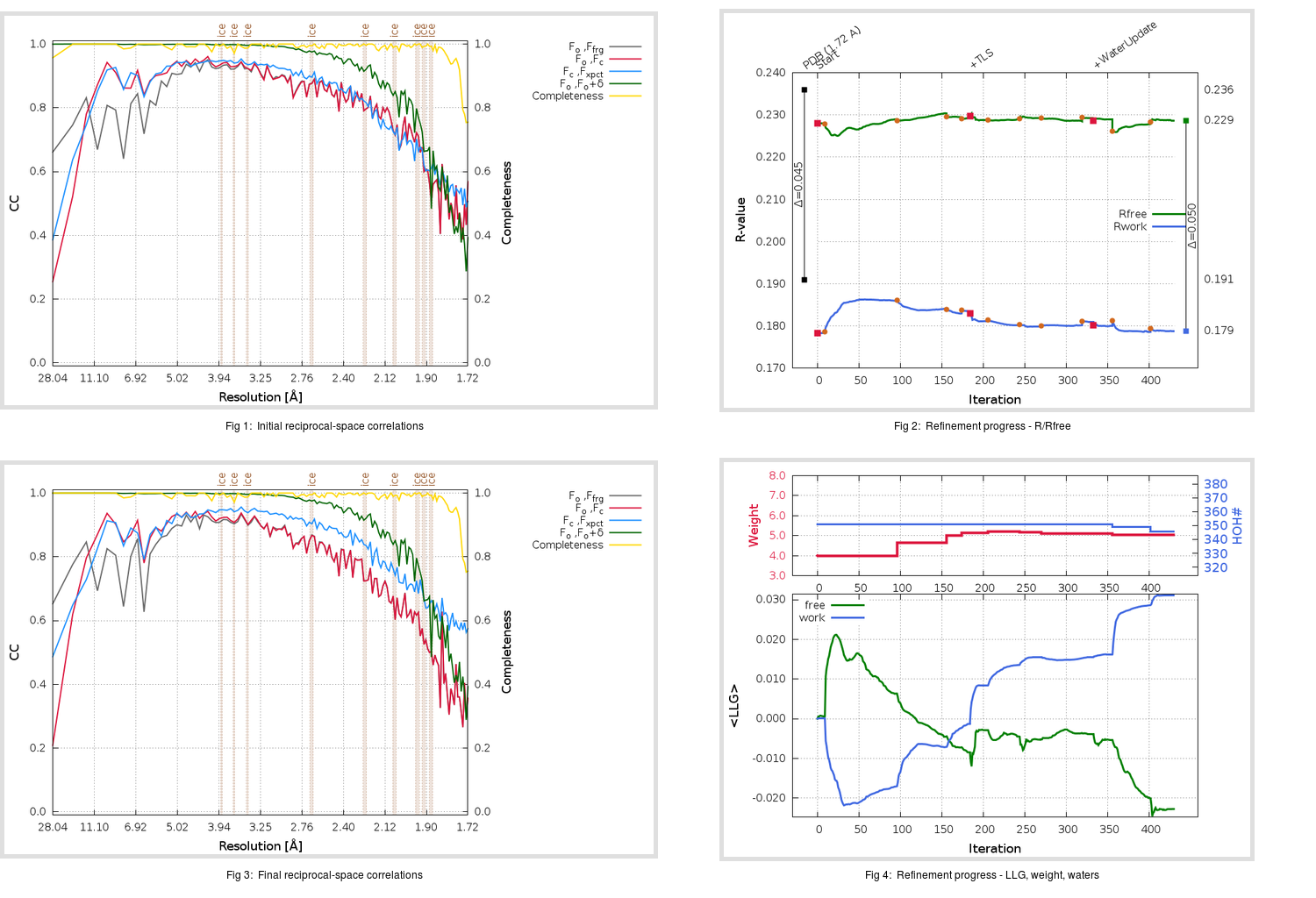Content:

## Deposited

` `
 Date deposited Date data collection Resolution R, Rfree 20200315 20200306 1.72 0.1880 0.2360

Molprobity (CCP4 7.0 version) summary:

```Ramachandran outliers =   0.33 %
favored =  96.03 %
Rotamer outliers      =   1.48 %
C-beta deviations     =     0
Clashscore            =   5.38
RMS(bonds)            =   0.0128
RMS(angles)           =   1.84
MolProbity score      =   1.69
Resolution            =   1.72
R-work                =   0.1880
R-free                =   0.2360
```

```Number of waters      =   351

<B> (all atoms) =   27.55 ( sd =    9.83 ) for       2795 non-hydrogen atoms
<B>   (protein) =   26.35 ( sd =    9.30 ) for       2416 non-hydrogen atoms
<B>     (water) =   35.42 ( sd =   10.54 ) for        351 non-hydrogen atoms
<B>    (others) =   37.31 ( sd =    9.36 ) for         28 non-hydrogen atoms

B min/max       (all non-hydrogen atoms) =   14.82 /   73.74
B min/max   (protein non-hydrogen atoms) =   14.82 /   73.74
B min/max     (water non-hydrogen atoms) =   15.07 /   71.29
B min/max     (other non-hydrogen atoms) =   26.77 /   49.07
```

## BUSTER (re-)refinement

` `

Molprobity (CCP4 7.0 version) summary:

```Ramachandran outliers =   0.33 %
favored =  98.01 %
Rotamer outliers      =   0.74 %
C-beta deviations     =     0
Clashscore            =   2.48
RMS(bonds)            =   0.0114
RMS(angles)           =   1.57
MolProbity score      =   1.03
Resolution            =   1.72
R-work                =   0.1789
R-free                =   0.2287
```

```Number of waters      =   346

<B> (all atoms) =   29.44 ( sd =    8.88 ) for       2790 non-hydrogen atoms
<B>   (protein) =   28.04 ( sd =    7.77 ) for       2416 non-hydrogen atoms
<B>     (water) =   38.80 ( sd =   10.79 ) for        346 non-hydrogen atoms
<B>    (others) =   38.03 ( sd =   11.38 ) for         28 non-hydrogen atoms

B min/max       (all non-hydrogen atoms) =    6.53 /   67.74
B min/max   (protein non-hydrogen atoms) =   17.29 /   67.45
B min/max     (water non-hydrogen atoms) =    6.53 /   67.74
B min/max     (other non-hydrogen atoms) =   26.71 /   52.78
```

Refinement progression:Results:

` `
 File Remark 5RG0_aB_refine.01_03_refine.pdb.gz exact refinement commands are in header 5RG0_aB_refine.01_03_refine.mtz.gz including original deposited data and several re-refinement map coefficients 5RG0_aB_refine.01_03_BUSTER_model.cif.gz including any non-standard compound restraints 5RG0_aB_refine.01_03_BUSTER_refln.cif.gz